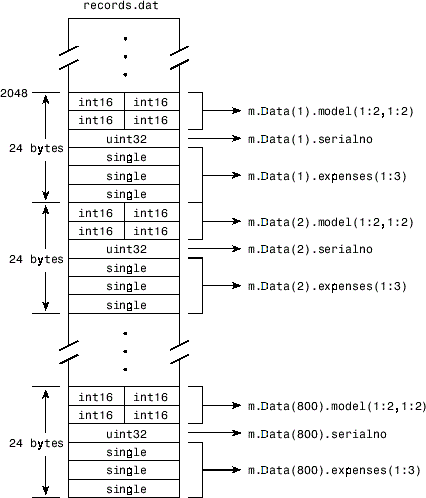Main Content

## Map File to Memory

### Create a Simple Memory Map

Suppose you want to create a memory map for a file named `records.dat`, using the `memmapfile` function.

Create a sample file named `records.dat`, containing 5000 values.

```rng('default') myData = rand([5000,1]); fileID = fopen('records.dat','w'); fwrite(fileID, myData,'double'); fclose(fileID);```

Next, create the memory map. Use the `Format` name-value pair argument to specify that the values are of type `double`. Use the `Writable` name-value pair argument to allow write access to the mapped region.

```m = memmapfile('records.dat', ... 'Format', 'double', ... 'Writable', true)```
```m = Filename: 'd:\matlab\records.dat' Writable: true Offset: 0 Format: 'double' Repeat: Inf Data: 5000x1 double array```

MATLAB® creates a `memmapfile` object, `m`. The `Format` property indicates that read and write operations to the mapped region treat the data in the file as a sequence of double-precision numbers. The `Data` property contains the 5000 values from the file, `records.dat`. You can change the value of any of the properties, except for `Data`, after you create the memory map, `m`.

For example, change the starting position of the memory map, `m`. Begin the mapped region 1024 bytes from the start of the file by changing the value of the `Offset` property.

`m.Offset = 1024`
```m = Filename: 'd:\matlab\records.dat' Writable: true Offset: 1024 Format: 'double' Repeat: Inf Data: 4872x1 double array```

Whenever you change the value of a memory map property, MATLAB remaps the file to memory. The `Data` property now contains only 4872 values.

### Specify Format of Your Mapped Data

By default, MATLAB considers all the data in a mapped file to be a sequence of unsigned 8-bit integers. However, your data might be of a different data type. When you call the `memmapfile` function, use the `Format` name-value pair argument to indicate another data type. The value of `Format` can either be a character vector that identifies a single class used throughout the mapped region, or a cell array that specifies more than one class.

Suppose you map a file that is 12 kilobytes in length. Data read from this file can be treated as a sequence of 6,000 16-bit (2-byte) integers, or as 1,500 8-byte double-precision floating-point numbers, to name just a few possibilities. You also could read this data as a combination of different types: for example, as 4,000 8-bit (1-byte) integers followed by 1,000 64-bit (8-byte) integers. You can determine how MATLAB will interpret the mapped data by setting the `Format` property of the memory map when you call the `memmapfile` function.

MATLAB arrays are stored on disk in column-major order. The sequence of array elements is column 1, row 1; column 1, row 2; column 1, last row; column 2, row 1, and so on. You might need to transpose or rearrange the order of array elements when reading or writing via a memory map.

### Map Multiple Data Types and Arrays

If the region you are mapping comprises segments of varying data types or array shapes, you can specify an individual format for each segment. Specify the value of the `Format` name-value pair argument as an `n`-by-3 cell array, where `n` is the number of segments. Each row in the cell array corresponds to a segment. The first cell in the row identifies the data type to apply to the mapped segment. The second cell contains the array dimensions to apply to the segment. The third cell contains the field name for referencing that segment. For a memory map, `m`, use the following syntax:

```m = memmapfile(filename, ... 'Format', { ... datatype1, dimensions1, fieldname1; ... datatype2, dimensions2, fieldname2; ... : : : ... datatypeN, dimensionsN, fieldnameN})```

Suppose you have a file that is 40,000 bytes in length. The following code maps the data beginning at the 2048th byte. The `Format` value is a 3-by-3 cell array that maps the file data to three different classes: `int16`, `uint32`, and `single`.

```m = memmapfile('records.dat', ... 'Offset', 2048, ... 'Format', { ... 'int16' [2 2] 'model'; ... 'uint32' [1 1] 'serialno'; ... 'single' [1 3] 'expenses'});```

In this case, `memmapfile` maps the `int16` data as a 2-by-2 matrix that you can access using the field name, `model`. The `uint32` data is a scalar value accessed using the field name, `serialno`. The `single` data is a 1-by-3 matrix named `expenses`. Each of these fields belongs to the 800-by-1 structure array, `m.Data`.

This figure shows the mapping of the example file.The next figure shows the ordering of the array elements more closely. In particular, it illustrates that MATLAB arrays are stored on the disk in column-major order. The sequence of array elements in the mapped file is row 1, column 1; row 2, column 1; row 1, column 2; and row 2, column 2.If the data in your file is not stored in this order, you might need to transpose or rearrange the order of array elements when reading or writing via a memory map.

### Select File to Map

You can change the value of the `Filename` property at any time after constructing the `memmapfile` object. You might want to do this if:

• You want to use the same `memmapfile` object on more than one file.

• You save your `memmapfile` object to a MAT-file, and then later load it back into MATLAB in an environment where the mapped file has been moved to a different location. This requires that you modify the path segment of the `Filename` to represent the new location.

Update the path in the `Filename` property for a memory map using dot notation. For example, to specify a new path, `f:\testfiles\records.dat` for a memory map, `m`, type:

`m.Filename = 'f:\testfiles\records.dat'`

Download ebook